Test: Quantitative Techniques- 4

# Test: Quantitative Techniques- 4

Test Description

## 20 Questions MCQ Test Mock Test Series for CLAT | Test: Quantitative Techniques- 4

Test: Quantitative Techniques- 4 for Banking Exams 2023 is part of Mock Test Series for CLAT preparation. The Test: Quantitative Techniques- 4 questions and answers have been prepared according to the Banking Exams exam syllabus.The Test: Quantitative Techniques- 4 MCQs are made for Banking Exams 2023 Exam. Find important definitions, questions, notes, meanings, examples, exercises, MCQs and online tests for Test: Quantitative Techniques- 4 below.
Solutions of Test: Quantitative Techniques- 4 questions in English are available as part of our Mock Test Series for CLAT for Banking Exams & Test: Quantitative Techniques- 4 solutions in Hindi for Mock Test Series for CLAT course. Download more important topics, notes, lectures and mock test series for Banking Exams Exam by signing up for free. Attempt Test: Quantitative Techniques- 4 | 20 questions in 30 minutes | Mock test for Banking Exams preparation | Free important questions MCQ to study Mock Test Series for CLAT for Banking Exams Exam | Download free PDF with solutions
 1 Crore+ students have signed up on EduRev. Have you?
Test: Quantitative Techniques- 4 - Question 1

### 2 / 3 is a rational number whereas √2 / √3 is

Test: Quantitative Techniques- 4 - Question 2

### Find the greatest number which divides 285 and 1249, leaving remainder 9 and 7?

Detailed Solution for Test: Quantitative Techniques- 4 - Question 2

Clearly required no is 138

Test: Quantitative Techniques- 4 - Question 3

### Shopkeeper offers 10% discount on an article and still makes a profit of 20%. What is the CP of the article marked at 500?

Detailed Solution for Test: Quantitative Techniques- 4 - Question 3

Marked price = 500
Discount = 10% of 500 = 450
S.P = 450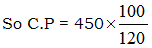= 375

Test: Quantitative Techniques- 4 - Question 4

A fair price shopkeeper takes 10% profit on his goods. He lost 20% goods during theft. His loss percent is?

Detailed Solution for Test: Quantitative Techniques- 4 - Question 4

Let cost price = 100
Loss during theft = 20
Saleable goods = 80
Profit taken = 10% of 80
= 8
S.P. = 88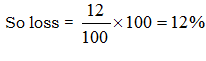Test: Quantitative Techniques- 4 - Question 5

If a diagonal of a rhombus are 8 and 6 then the square of its side is?

Detailed Solution for Test: Quantitative Techniques- 4 - Question 5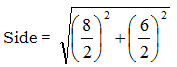= 5
(side)2 = 25

Test: Quantitative Techniques- 4 - Question 6

An angle in a semi-circle is?

Detailed Solution for Test: Quantitative Techniques- 4 - Question 6

The angle at the centre ∠AOB is twice the angle at the circumference ∠APB

As ∠AOB is 180°, it follows that ∠APB is 90°.

AOB is the diameter of the circle, so it follows that the angle in a semicircle is always a right angle: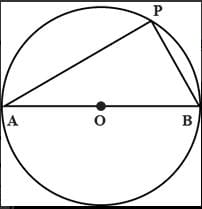Test: Quantitative Techniques- 4 - Question 7

Atyre has 2 punctures, the first can alone empty the tire in 9 min and 2nd can empty the tyre in 6 mins. How long will it take to empty the tyre by both punctures?

Detailed Solution for Test: Quantitative Techniques- 4 - Question 7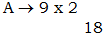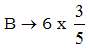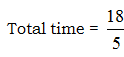Test: Quantitative Techniques- 4 - Question 8

In a group of 500 students, 103 students like coco cream and vanilla cream, 300 students like only vanilla cream how students like only coco cream?

Detailed Solution for Test: Quantitative Techniques- 4 - Question 8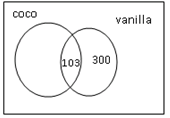So coco only = 500 – 300 – 103  = 97

Test: Quantitative Techniques- 4 - Question 9

(764 x?) ÷250= 382

Detailed Solution for Test: Quantitative Techniques- 4 - Question 9

(764 x ?) ÷ 250 = 382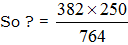= 125

Test: Quantitative Techniques- 4 - Question 10

285 is summation of 3 numbers. Ratio between 2nd and 3rd numbers is 6:5. Ratio between 1st and 2nd numbers is 3:7. The 3rd number is?

Detailed Solution for Test: Quantitative Techniques- 4 - Question 10 It is given that 1:2 = 3:7 and 2:3= 6:5
So 1:2:3=18:42:35
Third no.=35÷95×285
105
Test: Quantitative Techniques- 4 - Question 11

A box contains 36 marbles some are blue and others are green. If a marble is drawn at random from a box the probability that it is green is 2/3. Then no. of blue ballsare?

Detailed Solution for Test: Quantitative Techniques- 4 - Question 11

No. of blue balls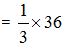= 12

Test: Quantitative Techniques- 4 - Question 12

Raman loses 30% of his money after spending 25% of the remaining he still has Rs 525. How much money did he originallyhad?

Detailed Solution for Test: Quantitative Techniques- 4 - Question 12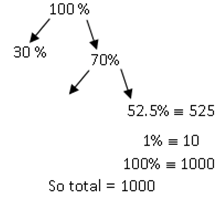Test: Quantitative Techniques- 4 - Question 13

If a point (x, y) ina oxy plane is equidistant from (-1, 1) and (4, 3) then?

Detailed Solution for Test: Quantitative Techniques- 4 - Question 13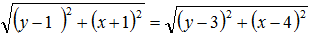x2 + y2 – 2y + 2x + 2 = y2 + x2 + 9 + 16 – 6y – 8x
10x + 4y = 23

Test: Quantitative Techniques- 4 - Question 14

15x ? - 8 x 2 – 9 = 0

Detailed Solution for Test: Quantitative Techniques- 4 - Question 14

15 x ? – 8 x 2 – 9 = 0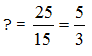Test: Quantitative Techniques- 4 - Question 15

If the diameter of a cylinder is 28 cm and its height is 20 cm, then total surface area is?

Detailed Solution for Test: Quantitative Techniques- 4 - Question 15

Total surface area = 2πr (r + h)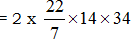= 2992

Test: Quantitative Techniques- 4 - Question 16

If the curved surface area of a cylinder is 1760 cm2 and its base radius is 14 cm when it’s height is?

Detailed Solution for Test: Quantitative Techniques- 4 - Question 16

Curved surface area = 1760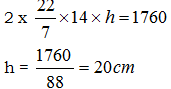Test: Quantitative Techniques- 4 - Question 17

Tap A and B can separately fill the tank in 10 hours 15 hours respectively. If both taps are opened together, how much time will it take to fill the tank fully?

Detailed Solution for Test: Quantitative Techniques- 4 - Question 17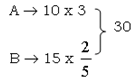So Time taked =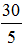= 6 hrs

Test: Quantitative Techniques- 4 - Question 18

On selling 100 mangoes, a person gains selling price of 20 mangoes. Find his gain percent?

Detailed Solution for Test: Quantitative Techniques- 4 - Question 18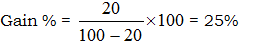Test: Quantitative Techniques- 4 - Question 19

The diameter of a wheel is 98cm. Howmuch revolutions will it take to cover a distance of 1540m?

Detailed Solution for Test: Quantitative Techniques- 4 - Question 19

Distance covered in one revolution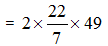= 308 cm
Total revolution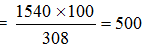Test: Quantitative Techniques- 4 - Question 20

Angles ofan quadrilateral are in the ratio 3:4:5:8. The smallest angle is?

Detailed Solution for Test: Quantitative Techniques- 4 - Question 20

3x + 4x + 5x + 8x = 360
20x = 360
x = 18
So smallest angles = 18 x 3 = 54°

## Mock Test Series for CLAT

2 videos|9 docs|77 tests
 Use Code STAYHOME200 and get INR 200 additional OFF Use Coupon Code
Information about Test: Quantitative Techniques- 4 Page
In this test you can find the Exam questions for Test: Quantitative Techniques- 4 solved & explained in the simplest way possible. Besides giving Questions and answers for Test: Quantitative Techniques- 4, EduRev gives you an ample number of Online tests for practice

## Mock Test Series for CLAT

2 videos|9 docs|77 tests